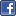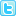# Abstract

## Effects of an Exercise Intervention on Body Composition in Older Adult Males Diagnosed with Parkinson√¢??s disease

As individuals grow, size of the body (height and weight) and specific organs increase. Different segments of the body and components of body mass, however, vary in the tempo and timing of growth. For example, the brain and the head will have already reached approximately 50% the mature size by about two years of age, while mature size is usually attained between 8 and 10 years of age. In contrast, the reproductive organs are later in growth reaching only 10% (in absolute terms) of adult size by the ages of 10 to 12 years. Puberty is characterized by accelerated tempo of growth evident in the growth spurt in height and associated increases in body size and mass, and also in the growth of the reproductive organs. Physiological functions are routinely expressed relative to stature or body mass. For example, peak oxygen uptake is commonly reported in millilitres per minute per unit body mass (ml kg-1 min-1). Fat-free mass, body surface area and stature are also used as denominators. However, issues related to the validity of estimates of fat free mass and body surface area need to be considered if these variables are used as denominators. For example, 54% and 52% of the variance in anaerobic mean and peak power obtained with the Wingate test (WAnT) was predicted by biological maturation, body mass and leg length in Portuguese adolescent basketball players aged 14-16 years. Biological maturation was expressed as the ratio of skeletal age (SA) to chronological age (CA) . The WAnT performance is often reported in Watt per unit of body mass or per unit of thigh volume. Ratio standards, isometry and elastic similarity Simple ratio standards (mL kg-1 min-1; W kg-1; W L-1) have been strongly criticised [2,3]. Expressing functions relative to anthropometric dimensions is meant to control for inter-individual variability in body size and is based on the assumption of geometric similarity. The ratios rely on the assumption that variables expressed as the ratio are linearly related, but the assumption can be erroneous. Although ratio standards are commonly used to control for the effects of body size on performance, other methods provide plausible alternatives. Relationships among length, surface area and volume have implications for metabolism and thermoregulation. All linear anthropometric dimensions of the body, such as stature, segment lengths, and breadths have the dimension d. All areas including body surface area and muscle cross-sectional areas have the dimension d2 . Total body volume given by air displacement plethysmography and other volumes (lung, heart, lower limb) have the dimension d3 . According to the second Newtonian law, time has the dimension d1 . Thus, peak oxygen uptake measured as volume per unit time (L min-1) should be proportional to d3 -d1 . In order to dissociate peak oxygen uptake from body size given by stature (m), values should be expressed in m2 . In isometric bodies stature corresponds to mass raised to 2/3 power function (assumption of geometric similarity) and an alternative denominator for peak oxygen uptake would be mL kg-0.67 min-1. However, a scaling exponent of mass rose to the power 0.75 (stature2.25) has also been suggested. Observations suggest that metabolic rate in many species of homeotherms conforms to mass raised to the power of 0.75 and not to the expected surface law of mass raised to the power of 0.67. A rationale for this apparent departure from theoretical predictions was offered by a model of elastic similarity . This model using a 0.75 exponent was proposed in zoology, but has been questioned as a statistical artefact because it was based on a single allometric model applied to data for a number of different species. When the data from specific species was interpreted separately, it had an intra-specific exponent of 0.67. These observations raise another question: how valid is the method of extrapolating an exponent for resting oxygen uptake in calculating maximal oxygen uptake? Assumptions for ratio standard, fundamentals of power functions and proportional allometric modelling Performance and physiological data often have a normal distribution. If the data have longer tail to the right side of the distribution, the data are described as positively skewed. In contrast, if the longer tail occurs on the left side of the distribution, the data are described as negatively skewed. Certain transformations can be used to overcome the problems of skewed data to correct the asymmetry and provide a normal distribution. For example, a log transformation will frequently correct positively skewed data such as body mass (kg) or maximal oxygen uptake (L min–1) into a ‘log-normal’ distribution. The Shapiro-Wilk test (SPSS) indicates whether the data fit a normal distribution. A ratio standard is valid only when the product-moment correlation coefficient between variables X and Y corresponds to coefficient of variation of X (standard deviation of X divided by the mean of X) divided by the coefficient of variation of Y (standard deviation of X divided by the mean of X). Allometric models present an alternative to ratio standards in assessing the effects of size-related increments on performance. An example of the allometric model is presented in eq. (1), where a is the

Author(s):

Corey A Peacock

Abstract | PDF

Share this###### Citations : 98

Journal of Clinical & Experimental Orthopaedics received 98 citations as per google scholar report